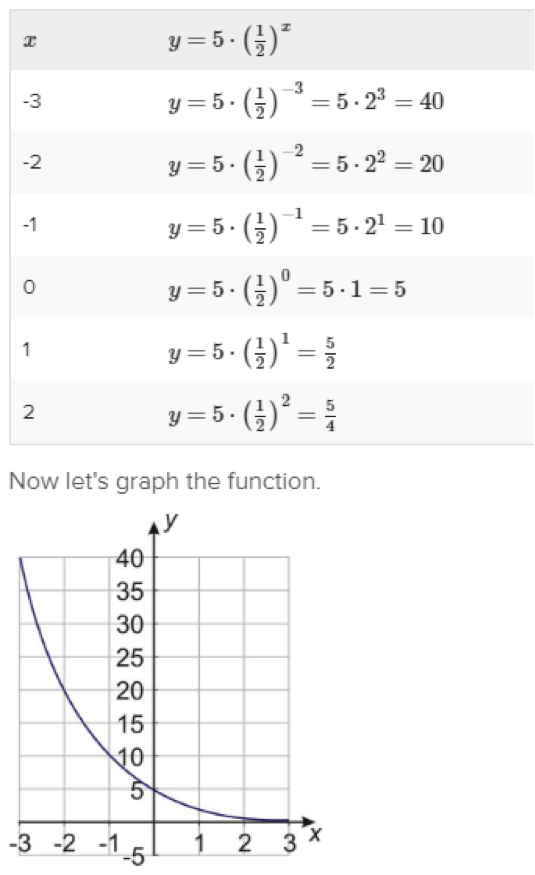# FNGR 1 | Lesson 1 | Explore (Exponential Growth/Decay)Below are examples of exponential growth, in the first two examples and an example of exponential decay.  What do you notice and what do you wonder?  Can you think of a real life example of exponential growth and one of exponential decay?

Examples of Growth:Example of Exponential Decay: